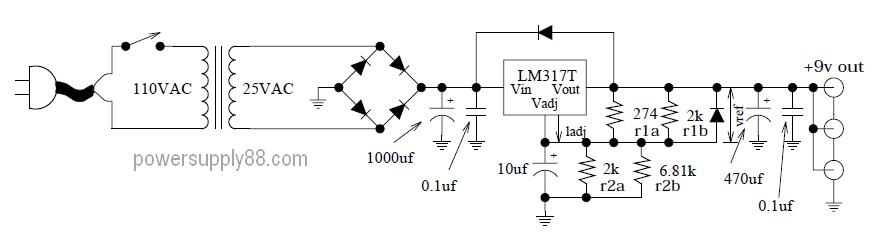# Super-Clean 9VDC Power Supply CircuitThis circuit will give you a 9V regulated DC voltage at maximum current of about 1A. The circuit uses stepdown tansformer with secondary output voltage of 25V AC and 1A current. The primary voltage depended your home electrical power line installation. If your power line voltage is 110V then you must find transformer 110V primary voltage. The diode can be 4 pieces of 1N4002 (1A rectifier diode) or a 1A bridge diode.

```Circuit notes:
274    2k
R1   = r1a || r1b = 241 ohms
R2   = r2a || r2b = 1456 ohms
Vref = 1.25V